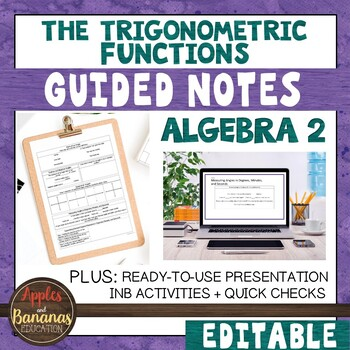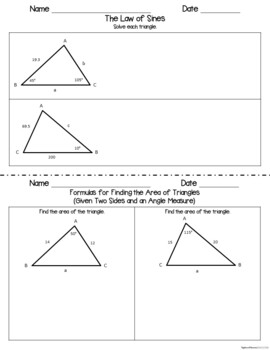# The Trigonometric Functions - Guided Notes and INB ActivitiesSubject
Resource Type
File Type
Zip (3 MB|47 pages)
Standards
\$6.20
List Price:
\$7.75
You Save:
\$1.55
Also included in:
1. This HUGE (over 550 pages) Algebra 2 bundle contains a full-year of focused, scaffolded notes and interactive notebook activities for 15 units of study, perfect for Algebra 2 students. There are over 195 guided note activities that contain sample teacher answer keys. Example problems are solved on
\$95.00
\$60.00
Save \$35.00
2. ********* If you have purchased our Algebra 2 Bundle you already have a lot of the material contained in this packet. We have a separate small bundle of the Pre-Calculus items that are not included in the Algebra 2 Bundle so that you can purchase the additional notes for Pre-Calc that are not includ
\$88.50
\$56.64
Save \$31.86
3. This HUGE (over 1,000 pages) high school bundle contains a full-year of guided/scaffolded notes and interactive notebook activities for an entire year of Algebra 1, Geometry, and Algebra 2. The Algebra 1 bundle contains 11 units of study plus 2 mini-units, the Geometry bundle contains 13 units, and
\$225.25
\$126.00
Save \$99.25
• Product Description
• Standards

Guided/scaffolded notes and interactive/foldable activities are perfect for Algebra 2, Pre-Calculus, or Integrated Math students. Focused notes help students to take clear and effective notes during math lessons, can be used for introducing new topics or for review, and can be used with any curriculum. The material is broken down in a way that is easily digestible for all types of math learners.

These math journal activities/scaffolded notes cover the trigonometric functions. The problems listed in this activity packet build nicely on each other if introduced in the order that they appear. Our students enjoy filling them out and organizing them in their math journals so that they can refer back to them throughout the year. These activities are a great way to promote writing in math. This packet also contains quick checks for understanding that can be used as an exit ticket or mini-quiz. Keys are included for reference. Please check out our full-length preview.

This set includes the following:

• Parts of an Angle (Match)
• Positive and Negative Angles
• Standard Position
• Measuring Angles in Degrees, Minutes, and Seconds
• Expressing Decimal Degree Measures in Degrees, Minutes, and Seconds
• Determine the Angle Measure of a Clockwise Rotation Around the Coordinate Plane
• Determine the Angle Measure of a Counterclockwise Rotation Around the Coordinate Plane
• Coterminal Angles and Reference Angles
• Using Quadrants to Figure out Reference Angles
• Find the Measure of the Reference Angle
• Review of the Pythagorean Theorem
• Find the Six Trigonometric Ratios for Angle A
• Trigonometric Ratios (Sine, Cosine, and Tangent)
• Reciprocal Trigonometric Ratios (Cosecant, Secant, and Cotangent)
• Special Right Triangle Relationships
• Trigonometric Ratios for 30°, 45°, and 60°
• Cofunctions
• Unit Circle on a Coordinate Plane
• The Six Trigonometric Functions on the Unit Circle
• Using the Unit Circle to Find Values of Trigonometric Functions
• Trigonometric Functions of an Angle in Standard Position
• Find the Values of the Six Trigonometric Functions for an Angle in Standard Position Given a Point on its Terminal Side
• Using Trigonometric Functions to Solve Problems Involving Right Triangles
• Frequently Used Values from the Unit Circle
• Inverses of Trigonometric Functions
• Solve an Equation Using the Inverse of Trigonometric Functions
• Evaluate an Expression with an Inverse Function Multiplied by a Function
• Solve a Right Triangle Using Trigonometric Ratios and the Pythagorean Theorem
• The Law of Sines
• Formulas for Finding the Area of Triangles (Given Two Sides and an Angle Measure)
• Formulas for Finding the Area of Triangles (Given Two Angles and a Side Measure)
• The Law of Cosines
• Heron’s Formula (a.k.a. Hero’s Formula)

Plus 13 Interactive Notebook activities

If your students need extra practice in this area, we recommend our The Trigonometric Functions Student Practice Pages product.

Check out our full-length preview for a closer look.

Related Products:

Scaffolded Notes/Interactive Notebook Bundles

Algebra 1 INB Bundle

Geometry INB Bundle

Algebra 2 INB Bundle

Pre-Calculus INB Bundle

Pre-Calculus Add on for Algebra 2 INB Bundle

Statistics INB Bundle

High School Math Interactive Notebook Bundle (Algebra 1, Geometry, Algebra 2)

Student Practice Pages Bundles

Algebra 1 Student Practice Pages Bundle

Geometry Student Practice Pages Bundle

Algebra 2 Student Practice Pages Bundle

High School Math Student Practice Pages Bundle (Algebra 1, Geometry, Algebra 2)

Assessment Bundles

Algebra 1 Assessment Bundle

**If you like what you see, please click on the "Follow Me" star to learn about new products, sales, and more!

Prove the Laws of Sines and Cosines and use them to solve problems.
Use special triangles to determine geometrically the values of sine, cosine, tangent for π/3, π/4 and π/6, and use the unit circle to express the values of sine, cosine, and tangent for π–𝘹, π+𝘹, and 2π–𝘹 in terms of their values for 𝘹, where 𝘹 is any real number.
Explain how the unit circle in the coordinate plane enables the extension of trigonometric functions to all real numbers, interpreted as radian measures of angles traversed counterclockwise around the unit circle.
Understand radian measure of an angle as the length of the arc on the unit circle subtended by the angle.
Total Pages
47 pages